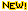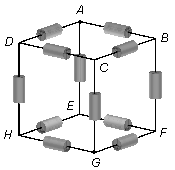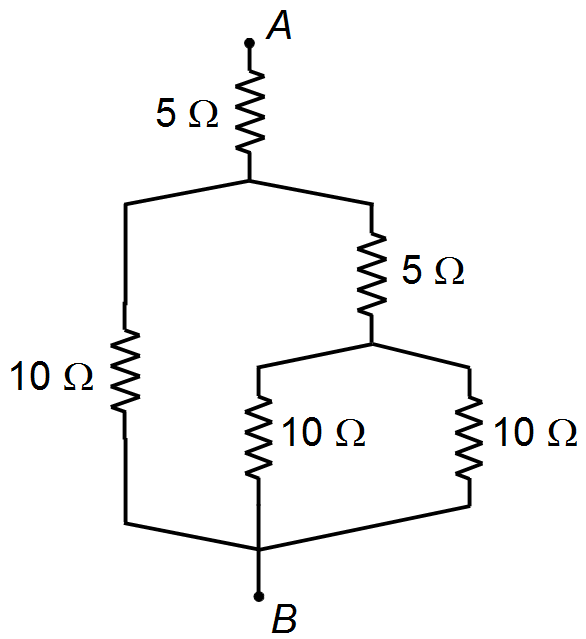ResistorsWe have 12 equal resistors with a value equal to R placed on the edges of a cube, as shown in the figure. Calculate the equivalent resistance between points A and G that form one of the main diagonals of the cube.Between the terminals, A and B of the figure, it is applied a voltage of 200 V. Calculate the magnitudes of the currents in each resistor and the equivalent resistance.